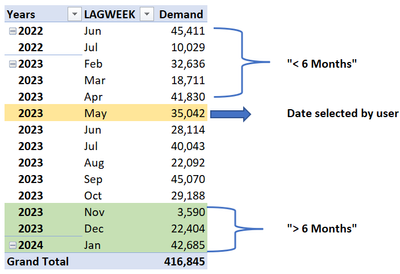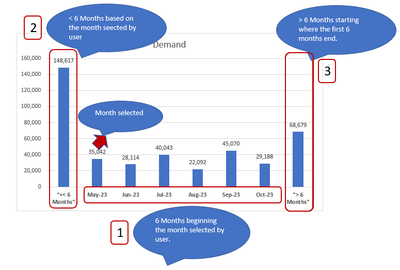cancel
Showing results for
Did you mean:Frequent Visitor

## How to format dynamic X axis in a Bar Chart

Hi all, I have a table with demand values in different dates and need a bar chart that changes the labels in x axis based on the date selected in a filter. I have attached Sample data.

Let's say the user selects a date in May 2023, so the bar chart should show the next 6 months of data and also show grouped in a label "> Months" the demand values for months after Oct-2023 and also it should group the previous 6 months of demand data with a label "< 6 months". The x axis labels of the chart should change based on the date selected.

Details of the logic if May-2023 is selected in a filter:Expected result:Is there a way to achieve it to have dynamic labels based on the selected date? I have tried with conditional columns but I am getting static results.

1 ACCEPTED SOLUTIONCommunity Support

Hi @JohanSmith7 ,

First create a new table for x-axis:

``````X-axis =
var _a = DISTINCT(SELECTCOLUMNS('Table',"YearMonth",FORMAT([Date],"MMM-YY"),"Rank",RANKX('Table',YEAR([Date])*100+MONTH([Date]),,ASC,Dense)))
var _b = COUNTROWS(_a)
return UNION(_a,{("> 6 months",_b),("< 6 months",0)})``````Then apply this measure to the chart:

``````Measure =
VAR _a =
MIN ( 'Table'[Date] )
VAR _b =
SELECTCOLUMNS (
CALENDAR ( _a, EDATE ( _a, 5 ) ),
"YearMonth", FORMAT ( [Date], "MMM-YY" )
) //6 months
VAR _c =
CALCULATE (
SUM ( 'Table'[Demand] ),
FILTER (
ALL ( 'Table' ),
FORMAT ( [Date], "MMM-YY" ) = SELECTEDVALUE ( 'X-axis'[YearMonth] )
)
) // calculate value for selected month
VAR _d =
CALCULATE ( SUM ( 'Table'[Demand] ), FILTER ( ALL ( 'Table' ), [Date] < _a ) ) //calculate value for < 6months
VAR _e =
CALCULATE (
SUM ( 'Table'[Demand] ),
FILTER ( ALL ( 'Table' ), [Date] > EOMONTH ( _a, 5 ) )
) //calculate value for > 6months
RETURN
SWITCH (
TRUE (),
SELECTEDVALUE ( 'X-axis'[YearMonth] ) IN _b,
CALCULATE (
SUM ( 'Table'[Demand] ),
FILTER (
ALL ( 'Table' ),
FORMAT ( [Date], "MMM-YY" ) = SELECTEDVALUE ( 'X-axis'[YearMonth] )
)
),
SELECTEDVALUE ( 'X-axis'[YearMonth] ) = "< 6 months", _d,
SELECTEDVALUE ( 'X-axis'[YearMonth] ) = "> 6 months", _e
)
``````Final output:Best Regards,

Jianbo Li

If this post helps, then please consider Accept it as the solution to help the other members find it more quickly.

3 REPLIES 3Community Support

Hi @JohanSmith7 ,

First create a new table for x-axis:

``````X-axis =
var _a = DISTINCT(SELECTCOLUMNS('Table',"YearMonth",FORMAT([Date],"MMM-YY"),"Rank",RANKX('Table',YEAR([Date])*100+MONTH([Date]),,ASC,Dense)))
var _b = COUNTROWS(_a)
return UNION(_a,{("> 6 months",_b),("< 6 months",0)})``````Then apply this measure to the chart:

``````Measure =
VAR _a =
MIN ( 'Table'[Date] )
VAR _b =
SELECTCOLUMNS (
CALENDAR ( _a, EDATE ( _a, 5 ) ),
"YearMonth", FORMAT ( [Date], "MMM-YY" )
) //6 months
VAR _c =
CALCULATE (
SUM ( 'Table'[Demand] ),
FILTER (
ALL ( 'Table' ),
FORMAT ( [Date], "MMM-YY" ) = SELECTEDVALUE ( 'X-axis'[YearMonth] )
)
) // calculate value for selected month
VAR _d =
CALCULATE ( SUM ( 'Table'[Demand] ), FILTER ( ALL ( 'Table' ), [Date] < _a ) ) //calculate value for < 6months
VAR _e =
CALCULATE (
SUM ( 'Table'[Demand] ),
FILTER ( ALL ( 'Table' ), [Date] > EOMONTH ( _a, 5 ) )
) //calculate value for > 6months
RETURN
SWITCH (
TRUE (),
SELECTEDVALUE ( 'X-axis'[YearMonth] ) IN _b,
CALCULATE (
SUM ( 'Table'[Demand] ),
FILTER (
ALL ( 'Table' ),
FORMAT ( [Date], "MMM-YY" ) = SELECTEDVALUE ( 'X-axis'[YearMonth] )
)
),
SELECTEDVALUE ( 'X-axis'[YearMonth] ) = "< 6 months", _d,
SELECTEDVALUE ( 'X-axis'[YearMonth] ) = "> 6 months", _e
)
``````Final output:Best Regards,

Jianbo Li

If this post helps, then please consider Accept it as the solution to help the other members find it more quickly.Community Support

Hi @JohanSmith7 ,

The number of months between 2023-May and 2022-Jun is greater than 6.
How to determine the date <6 months, is it based on the number of dates in your data?
Sorry for that the information you have provided is not making the problem clear to me. Can you please share more details to help us clarify your scenario?

Best Regards,

Jianbo Li

If this post helps, then please consider Accept it as the solution to help the other members find it more quickly.Frequent Visitor

Hi @v-jianboli-msft, yes the dates "< 6 months" and "> 6 months" should be determined based on the dates y my data.

I only have one column with dates "LAGWEEK".

Every time the user selects a date, the chart should:

1. Show 6 months of data beginning the month selected by the user.
2. Show < 6 Months --> this should sum data before the date selected by user.
3. Show > 6 Months--> this should sum data after the date selected. See the screenshot below:Hopefully the previous details helps to clarify what the chart should show,

Regards,